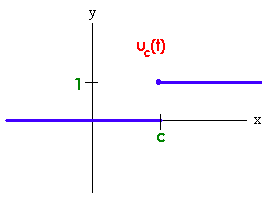$$\newcommand{\id}{\mathrm{id}}$$ $$\newcommand{\Span}{\mathrm{span}}$$ $$\newcommand{\kernel}{\mathrm{null}\,}$$ $$\newcommand{\range}{\mathrm{range}\,}$$ $$\newcommand{\RealPart}{\mathrm{Re}}$$ $$\newcommand{\ImaginaryPart}{\mathrm{Im}}$$ $$\newcommand{\Argument}{\mathrm{Arg}}$$ $$\newcommand{\norm}{\| #1 \|}$$ $$\newcommand{\inner}{\langle #1, #2 \rangle}$$ $$\newcommand{\Span}{\mathrm{span}}$$

# 6.8: Step Functions

$$\newcommand{\vecs}{\overset { \rightharpoonup} {\mathbf{#1}} }$$ $$\newcommand{\vecd}{\overset{-\!-\!\rightharpoonup}{\vphantom{a}\smash {#1}}}$$$$\newcommand{\id}{\mathrm{id}}$$ $$\newcommand{\Span}{\mathrm{span}}$$ $$\newcommand{\kernel}{\mathrm{null}\,}$$ $$\newcommand{\range}{\mathrm{range}\,}$$ $$\newcommand{\RealPart}{\mathrm{Re}}$$ $$\newcommand{\ImaginaryPart}{\mathrm{Im}}$$ $$\newcommand{\Argument}{\mathrm{Arg}}$$ $$\newcommand{\norm}{\| #1 \|}$$ $$\newcommand{\inner}{\langle #1, #2 \rangle}$$ $$\newcommand{\Span}{\mathrm{span}}$$ $$\newcommand{\id}{\mathrm{id}}$$ $$\newcommand{\Span}{\mathrm{span}}$$ $$\newcommand{\kernel}{\mathrm{null}\,}$$ $$\newcommand{\range}{\mathrm{range}\,}$$ $$\newcommand{\RealPart}{\mathrm{Re}}$$ $$\newcommand{\ImaginaryPart}{\mathrm{Im}}$$ $$\newcommand{\Argument}{\mathrm{Arg}}$$ $$\newcommand{\norm}{\| #1 \|}$$ $$\newcommand{\inner}{\langle #1, #2 \rangle}$$ $$\newcommand{\Span}{\mathrm{span}}$$

In this discussion, we will investigate piecewise defined functions and their Laplace Transforms. We start with the fundamental piecewise defined function, the Heaviside function.

Definition: The Heaviside Function

The Heaviside function, also called the unit step function, is defined by

u_c(t) = \left\{\begin{aligned} &1 && t <c \\ &0 && t \ge c \end{aligned} \right.

for $$c \ge 0$$.

The Heaviside function $$y = u_c(t)$$ and $$y = 1 - u_c(t)$$ are graphed below.Example $$\PageIndex{1}$$

We can write the function

f(t) = \left\{\begin{aligned} &3 && 0 \le x < 2 \\ &e^x && 2 \le x < 5 \\ &0 && 5 \le x \end{aligned} \right.

in terms of Heaviside functions.

Solution

We tackle the functions in parts. The function that is 1 from 0 to 2, and 0 otherwise is

$1 - u_2(x) .$

Multiplying by 3 gives

$3\left(1 - u_2(x)\right) = 3 - 3u_2(x) .$

To get the function that is 1 between 2 and 5 and 0 otherwise, we subtract

$u_2(x) - u_5(x) .$

Now multiply by $$e^x$$ to get

$e^x(u_2(x) - u_5(x)) = e^x u_2(x) - e^x u_5(x).$

\begin{align} f(x) &= 3 - 3u_2(x) + e^x\, u_2(x) - e^x \,u_5(x) \\ &= 3 + (e^x - 3)\,u_2(x) - e^x\, u_5(x) . \end{align}

We can find the Laplace transform of $$u_c(t)$$ by integrating

$\mathcal{L}\{u_c(t)\} = \int_0^{\infty} e^{st} u_c(t) \, dt$

$= \int _c^{\infty} e^{st} dt = \dfrac{e^{-cs}}{s}$

$L \{ u_c(t)\} = \dfrac{e^{-cs}}{s}.$

In practice, we want to find the Laplace transform of a more general piecewise defined function such as

f(t) = \left\{\begin{aligned} &0 && x < \pi \\ &\sin x && x \ge \pi \end{aligned} \right.

This type of function occurs in electronics when a switch is suddenly turned on after one second and a forcing function is applied. We can write

$f(x) = u_p(x) \sin x.$

We will be interested in the Laplace transform of a product of the Heaviside function with a continuous function. The result that we need is

$\mathcal{L}\{(u_c(t) f(t - c)\} = e^{-cs} \mathcal{L}\{f(t)\} .$

By taking inverses we get that if $$F(s) = \mathcal{L}\{f(t)\}$$, then

$L^{-1}\{e^{-cs}F(s)\} = u_c(t)f(t - c) .$

Proof

We use the definition to get

$\mathcal{L}\{u_c(t)f(t-c)\} = \int _0^{\infty} e^{-st}u_c(t)f(t-c)\,dt$

substitute $$\nu=t-c$$

$= \int _c^{\infty} e^{-st}f(t-c)\,dt = \int _0^{\infty} e^{-(\nu +c)s}f(\nu)\,d\nu$

$e^{-cs}\int _0^{\infty} e^{-s\nu}f(\nu)\,d\nu = e^{-cs}F(s).$

Example $$\PageIndex{2}$$

Find the Laplace transform of

f(t) = \left\{\begin{aligned} &0 && x < \pi \\ &\sin x && x \ge \pi. \end{aligned} \right.

Solution

We use that fact that

$f(x) = u_p(x) \sin x = -u_p(x) \sin[(x - p)] .$

Now we can use the formula to get that

$\mathcal{L}\{f(x)\} = -\mathcal{L}\{u_p(x) \sin[(x - p)]\} = -e^{-cs} \mathcal{L}\{\sin x\}.$

By the table of Laplace Transforms, we get

$= \dfrac{-e^{-cs}}{s^2 + 1} .$